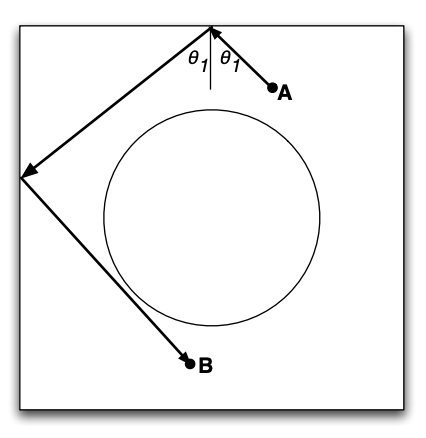시간 제한 메모리 제한 제출 정답 맞은 사람 정답 비율
1 초 128 MB 0 0 0 0.000%

## 문제

There are many similarities between the human and the Navi cultures and lifestyles. For instance, their sports and boardgames bear similarities to different sports and games on Earth. But at the same time, there are significant differences (e.g., instead of water polo, they play air polo on their Banshees). There is also a version of Billiards, which is quite similar to our version, but with the difference that their Billiards table has a large circular hole right at the center (see figure). Of course, a person who hits the ball into that hole immediately loses.

Jake and Tsu’tey start a friendly game of Billiards which soon turns into a not-so-friendly game with possibly the leadership of the Omaticaya at stake. In each round, they take turns. At each turn, an impartial judge places the cue ball at one location on the table (call it point A) and another ball somewhere else (call it point B). The person whose turn it is, must hit the ball at point B from point A with minimum number of rebounds off the walls of the table (see figure).

Your goal is to help Jake win the game and retain his leadership (otherwise he will have to try to tame Toruk once again, and only so many times you can survive that). Given the input parameters, you are to find the minimum number of rebounds needed to get from point A to point B (or declare that it is not possible to do so). Assume the board is perfectly frictionless and the walls are infinitely elastic. So a ball hit at an angle θ1 will rebound in the opposite direction at exactly the same angle.

## 입력

The Billiards table is a square with dimensions 100 by 100. Assume that the center of the table (and hence the center of the hole at the middle) is at (0, 0). You are given the x and y coordinates of the points A and B, and also the radius of the hole in the middle (r). The first line of the input file is the number of test cases. Each line contains 5 integers: Ax, Ay, Bx, By, r, where (Ax, Ay) are the coordinates of point A, and (Bx, By) are the coordinates of point B. The value r denotes the radius. You can assume that neither point A nor point B are in the hole, and that r < 50.

## 출력

For each test case, you are to find the minimum number of rebounds needed to reach from point A to point B. If it is less than 10, output on a single line ”REBOUNDS” followed by the number of rebounds needed. If it is not possible to hit point B from point A in < 10 rebounds, output on a single line ”NOT POSSIBLE”.

## 예제 입력 1

2
16 -14 38 -27 13
21 44 -38 -17 38


## 예제 출력 1

REBOUNDS 2
NOT POSSIBLE


## 힌트